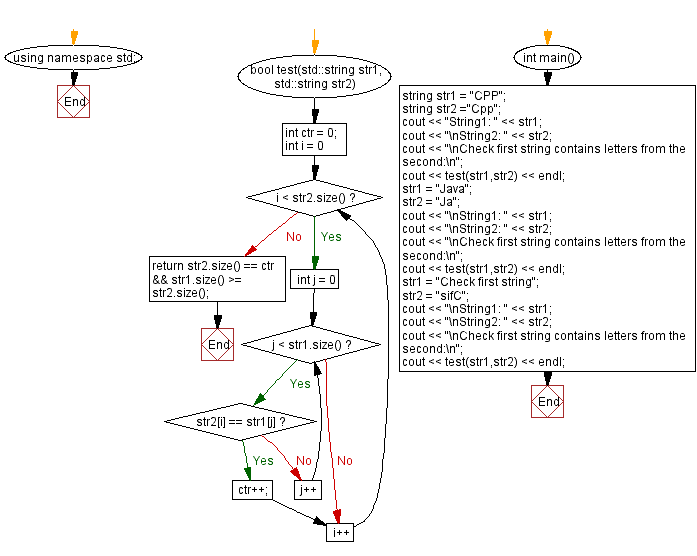﻿ C++ - Check first string contains letters from the second

# C++ String Exercises: Check first string contains letters from the second

## C++ String: Exercise-33 with Solution

A string is created using the letters of another string. Letters are case sensitive. Write a C++ program to verify that the letters in the second string appear in the first string. Return true otherwise false.

A string is created by using the letters of another string. Letters are case sensitive.

Test Data:
("CPP", "Cpp") -> false
("Java", "Ja") -> true
("Check first string", "sifC") ->true

Sample Solution:

C++ Code:

``````#include <bits/stdc++.h>
using namespace std;
bool test(std::string str1, std::string str2)
{
int ctr = 0;
for (int i = 0; i < str2.size(); i++)
{
for (int j = 0; j < str1.size(); j++)
{
if (str2[i] == str1[j])
{
ctr++;
break;
}
}
}
return str2.size() == ctr && str1.size() >= str2.size();
}

int main()
{
string str1 = "CPP";
string str2 ="Cpp";
cout << "String1: " << str1;
cout << "\nString2: " << str2;
cout << "\nCheck first string contains letters from the second:\n";
cout << test(str1,str2) << endl;
str1 = "Java";
str2 = "Ja";
cout << "\nString1: " << str1;
cout << "\nString2: " << str2;
cout << "\nCheck first string contains letters from the second:\n";
cout << test(str1,str2) << endl;
str1 = "Check first string";
str2 = "sifC";
cout << "\nString1: " << str1;
cout << "\nString2: " << str2;
cout << "\nCheck first string contains letters from the second:\n";
cout << test(str1,str2) << endl;
}
``````

Sample Output:

```String1: CPP
String2: Cpp
Check first string contains letters from the second:
0

String1: Java
String2: Ja
Check first string contains letters from the second:
1

String1: Check first string
String2: sifC
Check first string contains letters from the second:
1
```

Flowchart:C++ Code Editor:

Previous C++ Exercise: Reverse the words of three or more lengths in a string.

Next C++ Exercise: Remove a word from a given string.

What is the difficulty level of this exercise?

﻿

## C++ Programming: Tips of the Day

Why does the C++ map type argument require an empty constructor when using []?

This issue comes with operator[]. Quote from SGI documentation:

data_type&operator[](constkey_type& k) - Returns a reference to the object that is associated with a particular key. If the map does not already contain such an object, operator[] inserts the default object data_type().

If you don't have default constructor you can use insert/find functions. Following example works fine:

```myMap.insert(std::map<int, MyClass>::value_type ( 1, MyClass(1) ) );
myMap.find( 1 )->second;
```

Ref: https://bit.ly/3PQBRJi

We are closing our Disqus commenting system for some maintenanace issues. You may write to us at reach[at]yahoo[dot]com or visit us at Facebook